# Why Multiple Representations are Essential

Our calculus class on Tuesday was investigating derivatives of functions involving logarithms, particularly functions including natural logs. To begin our notes set, I worked a problem from algebra to help students remember how we use inverse operations to solve equations. I took direction from the students and solved the problem below in the following way.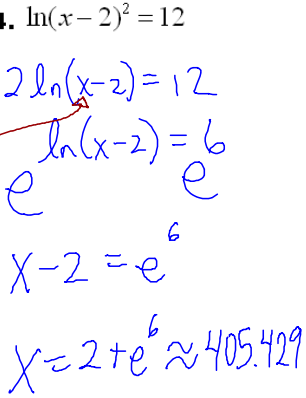I knew what would happen in advance - that students would simply go through the motions and apply algebraic properties to isolate x and move on. On a yearly basis, this is one of a set of problems I like to use to demonstrate to students the value of multiple representations. I then showed them the graph of the equation and how we could solve for the intersection of the logarithmic function and the constant function 12.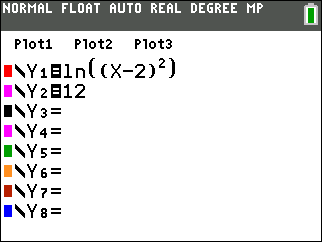We had an interesting discussion about the viewing window. Most students jumped all over "ZOOM-6", or Zoom --> Standard, the viewing window with x-axis and y-axis spanning the values -10 to 10 with units (tick marks) of 1.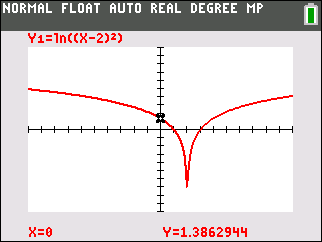Hmmmmm.... where's the 12? It's off the screen, of course!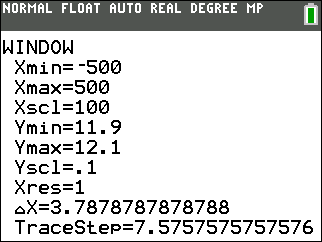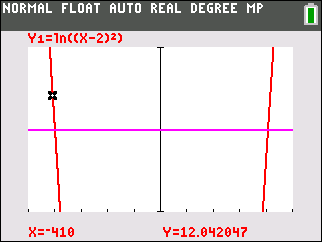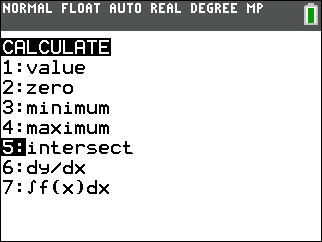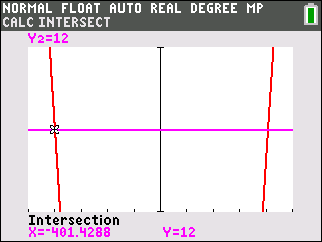After challenging their solution with the graphical argument, we decided to revisit our algebraic approach.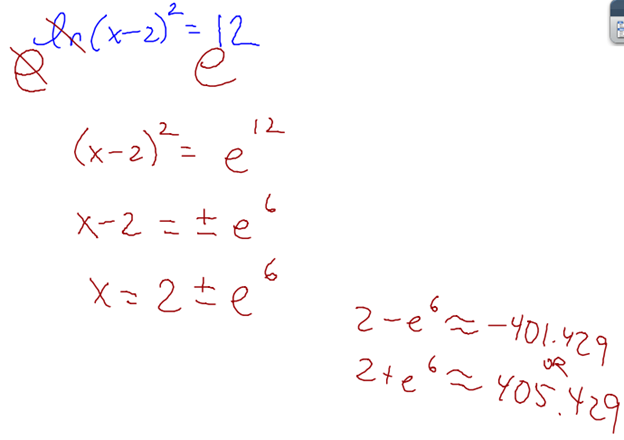This was a powerful way to convince the students that the exponent 2 acting on the quantity x - 2 has the effect of making the result positive, and it drove home the idea that we must consider the definition of absolute value when dealing with squares and square roots.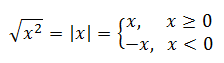We also had a good discussion about the original expression and whether the second power was acting on the quantity (x - 2), such as ln[ (x-2)*(x-2) ], or if it mattered if we consider ln[x-2]*ln[x-2].

Students often believe the plastic genie - the graphing calculator - knows all. I really enjoy the days in math class where I can show examples that disconfirm this student perception.

This site uses Akismet to reduce spam. Learn how your comment data is processed.